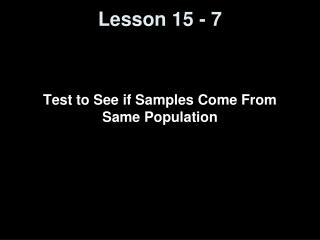DownloadDownload PresentationLesson 15 - 7

Lesson 15 - 7

Download PresentationLesson 15 - 7

- - - - - - - - - - - - - - - - - - - - - - - - - - - E N D - - - - - - - - - - - - - - - - - - - - - - - - - - -
Presentation Transcript

1. Lesson 15 - 7 Test to See if Samples Come From Same Population

2. Objectives • Test a claim using the Kruskal–Wallis test

3. Vocabulary • Kruskal–Wallis Test -- nonparametric procedure used to test the claim that k (3 or more) independent samples come from populations with the same distribution.

4. Test of Means of 3 or more groups • Parametric test of the means of three or more groups: • Compared the corresponding observations by subtracting one mean from the other • Performed a test of whether the mean is 0 • Nonparametric case for three or more groups: • Combine all of the samples and rank this combined set of data • Compare the rankings for the different groups

5. Kruskal-Wallis Test • Assumptions: • Samples are simple random samples from three or more populations • Data can be ranked • We would expect that the values of the samples, when combined into one large dataset, would be interspersed with each other • Thus we expect that the average relative ratings of each sample to be about the same

6. 12 R²1 R²2 R²k H = ------------- ----- + ----- + … + ------- - 3(N + 1) N(N + 1) n1 n2 nk Test Statistic for Kruskal–Wallis Test A computational formula for the test statistic is where Ri is the sum of the ranks of the ith sample R²1 is the sum of the ranks squared for the first sample R²2 is the sum of the ranks squared for the second sample, and so on n1 is the number of observations in the first sample n2 is the number of observations in the second sample, and so on N is the total number of observations (N = n1 + n2 + … + nk) k is the number of populations being compared. 12 1 ni(N + 1) ² H = -------------- --- Ri - ------------ N(N + 1) ni 2 Σ

7. Test Statistic (cont) • Large values of the test statistic H indicate that the Ri’s are different than expected • If H is too large, then we reject the null hypothesis that the distributions are the same • This always is a right-tailed test

8. Critical Value for Kruskal–Wallis Test Small-Sample Case When three populations are being compared and when the sample size from each population is 5 or less, the critical value is obtained from Table XIV in Appendix A. Large-Sample Case When four or more populations are being compared or the sample size from one population is more than 5, the critical value is χ²αwith k – 1 degrees of freedom, where k is the number of populations and αis the level of significance.

9. 12 R²1 R²2 R²k H = ------------- ----- + ----- + … + ------- - 3(N + 1) N(N + 1) n1 n2 nk Hypothesis Tests Using Kruskal–Wallis Test Step 0 Requirements: 1. The samples are independent random samples. 2. The data can be ranked. Step 1 Box Plots:Draw side-by-side boxplots to compare the sample data from the populations. Doing so helps to visualize the differences, if any, between the medians. Step 2 Hypotheses:(claim is made regarding distribution of three or more populations) H0: the distributions of the populations are the same H1: the distributions of the populations are not the same Step 3 Ranks:Rank all sample observations from smallest to largest. Handle ties by finding the mean of the ranks for tied values. Find the sum of the ranks for each sample. Step 4 Level of Significance:(level of significance determines the critical value) The critical value is found from Table XIV for small samples. The critical value is χ²αwith k – 1 degrees of freedom (found in Table VI) for large samples. Step 5 Compute Test Statistic: Step 6 Critical Value Comparison: We reject the null hypothesis if the test statistic is greater than the critical value.

10. Kruskal–Wallis Test Hypothesis • In this test, the hypotheses are H0: The distributions of all of the populations are the same H1: The distributions of all of the populations are not the same • This is a stronger hypothesis than in ANOVA, where only the means (and not the entire distributions) are compared

11. Example 1 from 15.7

12. 12 R²1 R²2 R²k H = ------------- ----- + ----- + … + ------- - 3(N + 1) N(N + 1) n1 n2 nk 12 194.5²225.5²246² H = ------------- ---------- + --------- + -------- - 3(36 + 1) = 1.009 36(36 + 1) 1212 12 Example 1 (cont) Critical Value: (Large-Sample Case) χ²αwith 2 (3 – 1) degrees of freedom, where 3 is the number of populations and 0.05 is the level of significance CV= 5.991 Conclusion: Since H < CV, therefore we FTR H0 (distributions are the same)

13. Summary and Homework • Summary • The Kruskal-Wallis test is a nonparametric test for comparing the distributions of three or more populations • This test is a comparison of the rank sums of the populations • Critical values for small samples are given in tables • The critical values for large samples can be approximated by a calculation with the chi-square distribution • Homework • problems 3, 5, 7, 10 from the CD

14. Homework Problem 3

15. Homework Problem5

16. Homework Problem 7

17. Homework Problem 10